# Algebra II : Radioactive Decay Equations

## Example Questions

2 Next →

### Example Question #321 : Exponents

Suppose 5 milligrams of element X decays to 3.2 milligrams after 48 hours.  What is the decay rate on a day to day basis?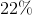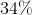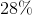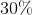Explanation:

Write the formula for radioactive decay.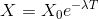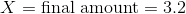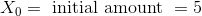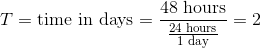Substitute the values in the equation and solve for lambda.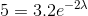Divide by 3.2 on both sides.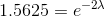Take the natural log of both sides to eliminate the exponential.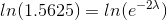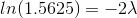Divide by negative two on both sides.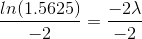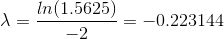Convert this to a percentage.

This element's decay rate is approximately:### Example Question #11 : Radioactive Decay Equations

An element has a half life of 6 days.  What is the approximate amount remaining for a 50 mg sample of this element after 5 days?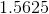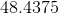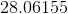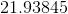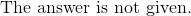Explanation:

Write the formula for half life.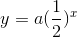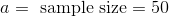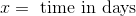Since the time requested is five out of the six day of the half life, the value ofis: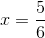Substitute all the known values into the equation.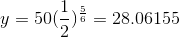The answer is:2 Next →

### All Algebra II Resources